﻿• 价格透明
• 信息保密
• 进度掌控
• 售后无忧# AI－常见机器学习算法介绍

## 常见机器学习算法名单

1. 线性回归
2. 逻辑回归
3. 决策树
4. SVM
5. 朴素贝叶斯
6. K最近邻算法
7. K均值算法
8. 随机森林算法
9. 降维算法

### 1、线性回归

• Y：因变量
• a：斜率
• x：自变量
• b ：截距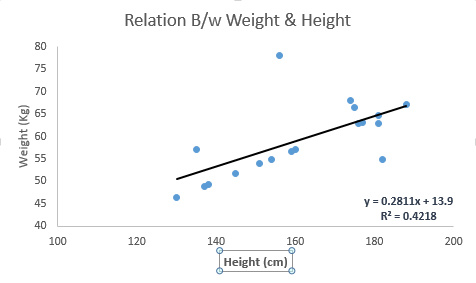Python 代码

 1 2 3 4 5 6 7 8 9 10 11 12 13 14 15 16 17 18 19 20 21 22 23 `#Import Library` `#Import other necessary libraries like pandas, numpy...` `from` ` sklearn ` `import` ` linear_model`   `#Load Train and Test datasets` `#Identify feature and response variable(s) and values must be numeric and numpy arrays` `x_train` `=` `input_variables_values_training_datasets` `y_train` `=` `target_variables_values_training_datasets` `x_test` `=` `input_variables_values_test_datasets`   `# Create linear regression object` `linear ` `=` ` linear_model.LinearRegression()`   `# Train the model using the training sets and check score` `linear.fit(x_train, y_train)` `linear.score(x_train, y_train)`   `#Equation coefficient and Intercept` `print` `(` `'Coefficient: n'` `, linear.coef_)` `print` `(` `'Intercept: n'` `, linear.intercept_)`   `#Predict Output` `predicted` `=` ` linear.predict(x_test)`

R代码

 1 2 3 4 5 6 7 8 9 10 11 12 13 `#Load Train and Test datasets` `#Identify feature and response variable(s) and values must be numeric and numpy arrays` `x_train <- input_variables_values_training_datasets` `y_train <- target_variables_values_training_datasets` `x_test <- input_variables_values_test_datasets` `x <- cbind(x_train,y_train)`   `# Train the model using the training sets and check score` `linear <- lm(y_train ~ ., data = x)` `summary(linear)`   `#Predict Output` `predicted= predict(linear,x_test)`

### 2、逻辑回归

 1 2 3 `odds= p/ (1-p) = probability of event occurrence / probability of not event occurrence` `ln(odds) = ln(p/(1-p))` `logit(p) = ln(p/(1-p)) = b0+b1X1+b2X2+b3X3....+bkXk`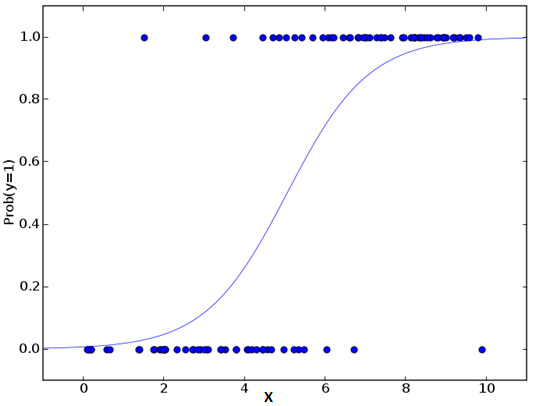Python代码

 1 2 3 4 5 6 7 8 9 10 11 12 13 14 15 16 `#Import Library` `from` ` sklearn.linear_model ` `import` ` LogisticRegression` `#Assumed you have, X (predictor) and Y (target) for training data set and x_test(predictor) of test_dataset` `# Create logistic regression object` `model ` `=` ` LogisticRegression()`   `# Train the model using the training sets and check score` `model.fit(X, y)` `model.score(X, y)`   `#Equation coefficient and Intercept` `print` `(` `'Coefficient: n'` `, model.coef_)` `print` `(` `'Intercept: n'` `, model.intercept_)`   `#Predict Output` `predicted` `=` ` model.predict(x_test)`

R代码

 1 2 3 4 5 6 7 `x <- cbind(x_train,y_train)` `# Train the model using the training sets and check score` `logistic <- glm(y_train ~ ., data = x,family='binomial')` `summary(logistic)`   `#Predict Output` `predicted= predict(logistic,x_test)`

• 加入交互项
• 精简模型特性
• 使用正则化方法
• 使用非线性模型

### 3、决策树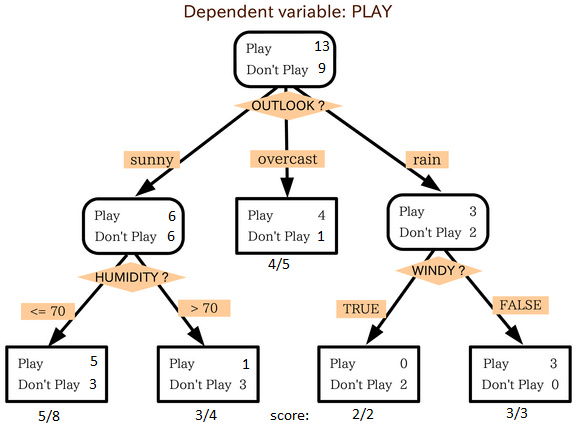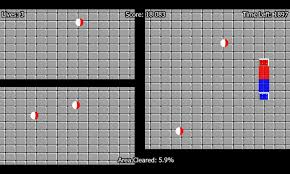Python代码

 1 2 3 4 5 6 7 8 9 10 11 12 13 14 15 `#Import Library` `#Import other necessary libraries like pandas, numpy...` `from` ` sklearn ` `import` ` tree`   `#Assumed you have, X (predictor) and Y (target) for training data set and x_test(predictor) of test_dataset` `# Create tree object` `model ` `=` ` tree.DecisionTreeClassifier(criterion` `=` `'gini'` `) ` `# for classification, here you can change the algorithm as gini or entropy (information gain) by default it is gini `   `# model = tree.DecisionTreeRegressor() for regression` `# Train the model using the training sets and check score` `model.fit(X, y)` `model.score(X, y)`   `#Predict Output` `predicted` `=` ` model.predict(x_test)`

R代码

 1 2 3 4 5 6 7 8 9 `library(rpart)` `x <- cbind(x_train,y_train)`   `# grow tree ` `fit <- rpart(y_train ~ ., data = x,method="class")` `summary(fit)`   `#Predict Output ` `predicted= predict(fit,x_test)`

### 4、支持向量机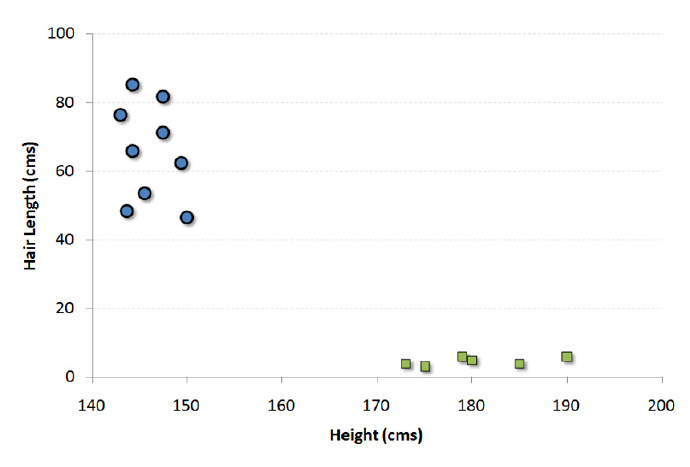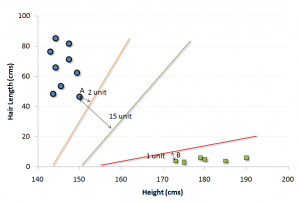上面示例中的黑线将数据分类优化成两个小组，两组中距离最近的点（图中A、B点）到达黑线的距离满足最优条件。这条直线就是我们的分割线。接下来，测试数据落到直线的哪一边，我们就将它分到哪一类去。

• 比起之前只能在水平方向或者竖直方向画直线，现在你可以在任意角度画线或平面。
• 游戏的目的变成把不同颜色的球分割在不同的空间里。
• 球的位置不会改变。

Python代码

 1 2 3 4 5 6 7 8 9 10 11 12 13 `#Import Library` `from` ` sklearn ` `import` ` svm`   `#Assumed you have, X (predic` `tor) ` `and` ` Y (target) ` `for` ` training data ` `set` ` and` ` x_test(predictor) of test_dataset` `# Create SVM classification object` `model ` `=` ` svm.svc() ` `# there is various option associated with it, this is simple for classification. You can refer link, for mo# re detail.` `# Train the model using the training sets and check score` `model.fit(X, y)` `model.score(X, y)`   `#Predict Output` `predicted` `=` ` model.predict(x_test)`

R代码

 1 2 3 4 5 6 7 8 9 `library(e1071)` `x <- cbind(x_train,y_train)`   `# Fitting model` `fit <-svm(y_train ~ ., data = x)` `summary(fit)`   `#Predict Output ` `predicted= predict(fit,x_test)`

### 5、朴素贝叶斯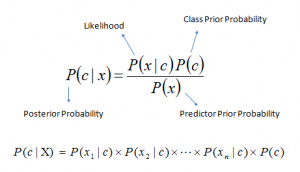• P(c|x) 是已知预示变量（属性）的前提下，类（目标）的后验概率
• P(c) 是类的先验概率
• P(x|c) 是可能性，即已知类的前提下，预示变量的概率
• P(x) 是预示变量的先验概率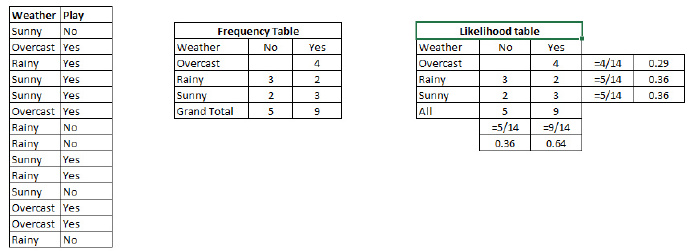Python代码

 1 2 3 4 5 6 7 8 9 10 `#Import Library` `from` ` sklearn.naive_bayes ` `import` ` GaussianNB`   `#Assumed you have, X (predictor) and Y (target) for training data set and x_test(predictor) of test_dataset` `# Create SVM classification object model = GaussianNB() # there is other distribution for multinomial classes like Bernoulli Naive Bayes, Refer link` `# Train the model using the training sets and check score` `model.fit(X, y)`   `#Predict Output` `predicted` `=` ` model.predict(x_test)`

R代码

 1 2 3 4 5 6 7 8 9 `library(e1071)` `x <- cbind(x_train,y_train)`   `# Fitting model` `fit <-naiveBayes(y_train ~ ., data = x)` `summary(fit)`   `#Predict Output ` `predicted= predict(fit,x_test)`

### 6、KNN（K – 最近邻算法）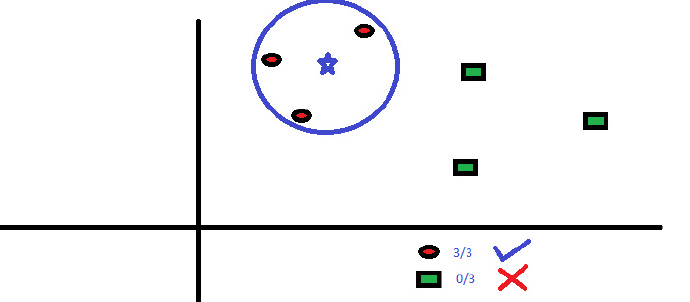• KNN 的计算成本很高。
• 变量应该先标准化（normalized），不然会被更高范围的变量偏倚。
• 在使用KNN之前，要在野值去除和噪音去除等前期处理多花功夫。

Python代码

 1 2 3 4 5 6 7 8 9 10 11 12 `#Import Library` `from` ` sklearn.neighbors ` `import` ` KNeighborsClassifier`   `#Assumed you have, X (predictor) and Y (target) for training data set and x_test(predictor) of test_dataset` `# Create KNeighbors classifier object model` `KNeighborsClassifier(n_neighbors` `=` `6` `) ` `# default value for n_neighbors is 5`   `# Train the model using the training sets and check score` `model.fit(X, y)`   `#Predict Output` `predicted` `=` ` model.predict(x_test)`

R代码

 1 2 3 4 5 6 7 8 9 `library(knn)` `x <- cbind(x_train,y_train)`   `# Fitting model` `fit <-knn(y_train ~ ., data = x,k=5)` `summary(fit)`   `#Predict Output ` `predicted= predict(fit,x_test)`

### 7、K 均值算法

K – 均值算法是一种非监督式学习算法，它能解决聚类问题。使用 K – 均值算法来将一个数据归入一定数量的集群（假设有 k 个集群）的过程是简单的。一个集群内的数据点是均匀齐次的，并且异于别的集群。K – 均值算法怎样形成集群：

1. K – 均值算法给每个集群选择k个点。这些点称作为质心。
2. 每一个数据点与距离最近的质心形成一个集群，也就是 k 个集群。
3. 根据现有的类别成员，找出每个类别的质心。现在我们有了新质心。
4. 当我们有新质心后，重复步骤 2 和步骤 3。找到距离每个数据点最近的质心，并与新的k集群联系起来。重复这个过程，直到数据都收敛了，也就是当质心不再改变。

K – 均值算法涉及到集群，每个集群有自己的质心。一个集群内的质心和各数据点之间距离的平方和形成了这个集群的平方值之和。同时，当所有集群的平方值之和加起来的时候，就组成了集群方案的平方值之和。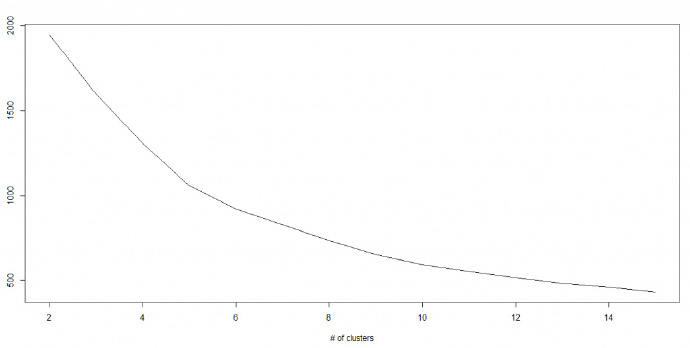Python代码

 1 2 3 4 5 6 7 8 9 10 11 12 `#Import Library` `from` ` sklearn.cluster ` `import` ` KMeans`   `#Assumed you have, X (attributes) for training data set and x_test(attributes) of test_dataset` `# Create KNeighbors classifier object model` `k_means ` `=` ` KMeans(n_clusters` `=` `3` `, random_state` `=` `0` `)`   `# Train the model using the training sets and check score` `model.fit(X)`   `#Predict Output` `predicted` `=` ` model.predict(x_test)`

R代码

 1 2 `library(cluster)` `fit <- kmeans(X, 3) # 5 cluster solution`

### 8、随机森林

1. 如果训练集的案例数是 N，则从 N 个案例中用重置抽样法随机抽取样本。这个样本将作为“养育”树的训练集。
2. 假如有 M 个输入变量，则定义一个数字 m<<M。m 表示，从 M 中随机选中 m 个变量，这 m 个变量中最好的切分会被用来切分该节点。在种植森林的过程中，m 的值保持不变。
3. 尽可能大地种植每一棵树，全程不剪枝。

1. 随机森林入门—简化版
2. 将 CART 模型与随机森林比较（上）
3. 将随机森林与 CART 模型比较（下）
4. 调整你的随机森林模型参数

Python

 1 2 3 4 5 6 7 8 9 10 11 12 `#Import Library` `from` ` sklearn.ensemble ` `import` ` RandomForestClassifier`   `#Assumed you have, X (predictor) and Y (target) for training data set and x_test(predictor) of test_dataset` `# Create Random Forest object` `model` `=` ` RandomForestClassifier()`   `# Train the model using the training sets and check score` `model.fit(X, y)`   `#Predict Output` `predicted` `=` ` model.predict(x_test)`

R代码

 1 2 3 4 5 6 7 8 9 `library(randomForest)` `x <- cbind(x_train,y_train)`   `# Fitting model` `fit <- randomForest(Species ~ ., x,ntree=500)` `summary(fit)`   `#Predict Output ` `predicted= predict(fit,x_test)`

### 9、降维算法

Python代码

 1 2 3 4 5 6 7 8 9 10 11 12 13 14 `#Import Library` `from` ` sklearn ` `import` ` decomposition`   `#Assumed you have training and test data set as train and test` `# Create PCA obeject pca= decomposition.PCA(n_components=k) #default value of k =min(n_sample, n_features)` `# For Factor analysis` `#fa= decomposition.FactorAnalysis()` `# Reduced the dimension of training dataset using PCA` `train_reduced ` `=` ` pca.fit_transform(train)`   `#Reduced the dimension of test dataset` `test_reduced ` `=` ` pca.transform(test)`   `#For more detail on this, please refer  this link.`

R Code

 1 2 3 4 `library(stats)` `pca <- princomp(train, cor = TRUE)` `train_reduced  <- predict(pca,train)` `test_reduced  <- predict(pca,test)`

Python代码

 1 2 3 4 5 6 7 8 9 10 11 12 `#Import Library` `from` ` sklearn.ensemble ` `import` ` GradientBoostingClassifier`   `#Assumed you have, X (predictor) and Y (target) for training data set and x_test(predictor) of test_dataset` `# Create Gradient Boosting Classifier object` `model` `=` ` GradientBoostingClassifier(n_estimators` `=` `100` `, learning_rate` `=` `1.0` `, max_depth` `=` `1` `, random_state` `=` `0` `)`   `# Train the model using the training sets and check score` `model.fit(X, y)`   `#Predict Output` `predicted` `=` ` model.predict(x_test)`

R代码

 1 2 3 4 5 6 7 `library(caret)` `x <- cbind(x_train,y_train)`   `# Fitting model` `fitControl <- trainControl( method = "repeatedcv", number = 4, repeats = 4)` `fit <- train(y ~ ., data = x, method = "gbm", trControl = fitControl,verbose = FALSE)` `predicted= predict(fit,x_test,type= "prob")[,2]`### 低价透明### 金牌服务### 信息保密